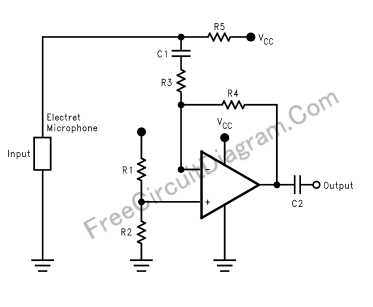# Battery Powered Electret Microphone Pre-AmplifierHere is a schematic diagram of electret microphone pre-amplifier using LMV721 op-amp. because the LMV721 has low noise and low power features, it would be an ideal choice for  battery powered microphone preamplifier. The LMV721 is connected as an inverting amplifier.Resistors, R1 =R2 = 4.7k, sets the reference at mid point between VCC = 3V and ground. Thus, this set the op amp for single supply use. The gain of this preamplifier, which is 34dB (50x), is set by resistors R3 = 10k and R4 = 500k. A 10 MHz gain bandwidth product for the LMV721 is more than enough for most audio application since the normal audio range is from 20 Hz to 20kHz. A resistor R5 =5k provides the bias voltage for the electret microphone. Capacitors C1 =C2 = 4.7µF at the input and output is provided to  block out the DC voltage offset. [Circuit schematic diagram source: National Semiconductor Application Notes]